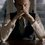# Help needed!!

I was just reading Feynman lectures and there was a series which came there. There its value was assumed. But I thought it must be having a value as it is converging. But it was really difficult to find a closed form. The series was -

$\displaystyle \sum_{k=0}^{\infty}{\frac{{(-1)}^{k}}{\sqrt{{x}^{2}+{k}^{2}}}}$

I learnt Fourier Transform and Poisson sum formula too but they are not helping me. Actually the topic was electrostatic energy of an ionic crystal.

Any type of help will be appreciated!Note by Kartik Sharma
6 years, 4 months ago

This discussion board is a place to discuss our Daily Challenges and the math and science related to those challenges. Explanations are more than just a solution — they should explain the steps and thinking strategies that you used to obtain the solution. Comments should further the discussion of math and science.

When posting on Brilliant:

• Use the emojis to react to an explanation, whether you're congratulating a job well done , or just really confused .
• Ask specific questions about the challenge or the steps in somebody's explanation. Well-posed questions can add a lot to the discussion, but posting "I don't understand!" doesn't help anyone.
• Try to contribute something new to the discussion, whether it is an extension, generalization or other idea related to the challenge.

MarkdownAppears as
*italics* or _italics_ italics
**bold** or __bold__ bold
- bulleted- list
• bulleted
• list
1. numbered2. list
1. numbered
2. list
Note: you must add a full line of space before and after lists for them to show up correctly
paragraph 1paragraph 2

paragraph 1

paragraph 2

[example link](https://brilliant.org)example link
> This is a quote
This is a quote
    # I indented these lines
# 4 spaces, and now they show
# up as a code block.

print "hello world"
# I indented these lines
# 4 spaces, and now they show
# up as a code block.

print "hello world"
MathAppears as
Remember to wrap math in $$ ... $$ or $ ... $ to ensure proper formatting.
2 \times 3 $2 \times 3$
2^{34} $2^{34}$
a_{i-1} $a_{i-1}$
\frac{2}{3} $\frac{2}{3}$
\sqrt{2} $\sqrt{2}$
\sum_{i=1}^3 $\sum_{i=1}^3$
\sin \theta $\sin \theta$
\boxed{123} $\boxed{123}$

Sort by:

Did you look up Ewald's summation ? As far as I know , fourier transform comes up in that summation only , and if that is indeed the case , sorry because I $\in [Novice^{+},Intermediate^{-}]$ !

- 6 years, 4 months ago

:D Interesting interval there.

- 6 years, 4 months ago

Haha yes!

- 6 years, 4 months ago

Well, it came in one of Pratik's questions as well and that question is quite a bit same as this one.

- 6 years, 4 months ago

- 6 years, 4 months ago